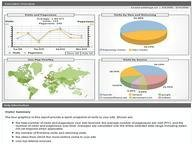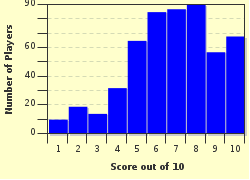FREE! Click here to Join FunTrivia. Thousands of games, quizzes, and lots more!# Mean, Mode and Median Trivia Quiz

### A few questions to test your knowledge of mean, mode and median which are used frequently in statistics. Enjoy!

A multiple-choice quiz by Matthew_07. Estimated time: 4 mins.

Author
Matthew_07
Time
4 mins
Type
Multiple Choice
Quiz #
289,559
Updated
Dec 03 21
# Qns
10
Difficulty
Average
Avg Score
7 / 10
Plays
1800
Last 3 plays: Guest 181 (8/10), Guest 89 (6/10), Quizaddict1 (5/10).
This quiz has 2 formats: you can play it as a or as shown below.
Scroll down to the bottom for the answer key.
1. In statistics, the mean is the sum of all the observations divided by the number of observations. In other words, the mean is the average value of a set of data. The mean of a set that consists of five data points is 20. You are also given that three of the five data points are 5, 15 and 30. The remaining two numbers share the same value, which is ___. Hint

20
25
35
40

#### NEXT>

2. There are many types of mean. The most common ones are arithmetic mean and geometric mean. Arithmetic means are calculated by first summing up all the individual data points, then dividing the summation by the number of observations. On the other hand, to find the geometric mean of a set of data, which two mathematical operations are involved? Hint

Multiplication and factorial
Subtraction and exponentiation
Subtraction and factorial
Multiplication and exponentiation

#### NEXT>

3. In mathematics, there is a statistical measure of the magnitude of a quantity which can take both positive and negative values. This measure is very useful for data points which are obtained from trigonometric functions. Its abbreviation is RMS. What does it stand for? Hint

Root mean summation
Relative mean summation
Relative mean square
Root mean square

#### NEXT>

4. In statistics, the mode refers to the value which occurs with the highest frequency in a set of data. For example, 2 is the mode for the set of data points 1, 1, 2, 2, 2, 2, 2, 3, since 2 occurs most frequently. Now, if we have a set of data which consists of 1, 1, 1, 2, 2, 2, 3, what is (are) the mode(s)? Hint

Both 1 and 2.
1.5
There is no mode.
2

#### NEXT>

5. You are given a bell-shaped Gaussian distribution graph which is a continuous probability distribution. Where can you find the mode? At the ___ of the graph. Hint

Left tail
Peak
Right tail

#### NEXT>

6. Unlike the arithmetic mean, the mode of a set of data is not unique (can have more than one value). What is the mode for the following data points? 1, 2, 3, 4, 5, 6, 7, 8, 9, 10. Hint

5.5
5
1, 2, 3,4 ,5 ,6 ,7 ,8 ,9, 10
There is no mode.

#### NEXT>

7. In statistics, the median of a set of data is more commonly known as the "middle" data. Let say we have 10, 20, 30, 40, 50. So, its median is 30. What is the median for the following set of data, which has an even number of observations? 10, 20, 30, 40, 50, 60. Hint

30
35
40
There is no median.

#### NEXT>

8. Before finding the median of a set of data, what is the crucial step that we need to do? Hint

Arrange the data points in ascending or descending order.
Check to see if there is any repeating data point.
Multiply each data point by 10.
Add 5 to each data point.

#### NEXT>

9. The alternative name(s) for the median is (are) ___. Hint

The 50th percentile
The second quartile
The fifth decile
All of these.

#### NEXT>

10. What is the relationship between the mean, the mode and the median for a standard normal distribution? Hint

Mean = mode = median
Mode < median < mean
Mean < median < mode
Median < mode < mean

 (Optional) Create a Free FunTrivia ID to save the points you are about to earn:Select a User ID:Choose a Password:Your Email:

Most Recent Scores
Sep 15 2023 : Guest 181: 8/10
Aug 31 2023 : Guest 89: 6/10
Aug 12 2023 : Quizaddict1: 5/10
Aug 07 2023 : Guest 86: 10/10

Score DistributionQuiz Answer Key and Fun Facts
1. In statistics, the mean is the sum of all the observations divided by the number of observations. In other words, the mean is the average value of a set of data. The mean of a set that consists of five data points is 20. You are also given that three of the five data points are 5, 15 and 30. The remaining two numbers share the same value, which is ___.

First, we need to find the sum of the five data points, which is 5 x 20 = 100. Then, we subtract 5, 15 and 30 from 100, which gives us 100 - 5 - 15 - 30 = 50. This 50 is the sum of the two unknown data points. We are given that the two unknown data points share the same value. So, 50 / 2 = 25, which is the answer we are looking for.
2. There are many types of mean. The most common ones are arithmetic mean and geometric mean. Arithmetic means are calculated by first summing up all the individual data points, then dividing the summation by the number of observations. On the other hand, to find the geometric mean of a set of data, which two mathematical operations are involved?

Let say we have three data points, which are 1, 3 and 9. The arithmetic mean is (1 + 3 + 9)/3 = 4.333. On the other hand, its geometric mean is (1 x 3 x 9)^(1/3) = 3.
3. In mathematics, there is a statistical measure of the magnitude of a quantity which can take both positive and negative values. This measure is very useful for data points which are obtained from trigonometric functions. Its abbreviation is RMS. What does it stand for?

Trigonometric functions give both positive values (above the x-axis) and negative values (below the x-axis). RMS (root mean square) gives a positive value which indicates the magnitude (distance) of a varying quantity.
4. In statistics, the mode refers to the value which occurs with the highest frequency in a set of data. For example, 2 is the mode for the set of data points 1, 1, 2, 2, 2, 2, 2, 3, since 2 occurs most frequently. Now, if we have a set of data which consists of 1, 1, 1, 2, 2, 2, 3, what is (are) the mode(s)?

When there are two numbers that occur with the same highest frequency, both of them are the modes of that set of data.
5. You are given a bell-shaped Gaussian distribution graph which is a continuous probability distribution. Where can you find the mode? At the ___ of the graph.

The Gaussian distribution is also known as the normal distribution. Since the mode is the data that occurs most frequently, therefore we can find the value at the peak of the graph.
6. Unlike the arithmetic mean, the mode of a set of data is not unique (can have more than one value). What is the mode for the following data points? 1, 2, 3, 4, 5, 6, 7, 8, 9, 10.

This is the problem when we use the mode as the measure of central tendency. In this case, there is no mode, since each data point occurs only once.
7. In statistics, the median of a set of data is more commonly known as the "middle" data. Let say we have 10, 20, 30, 40, 50. So, its median is 30. What is the median for the following set of data, which has an even number of observations? 10, 20, 30, 40, 50, 60.

There are 6 observations in the set of data. So, if we try to find the middle value, it is the value between 30 and 40. To find the median, we find the arithmetic mean for 30 and 40, which is (30 + 40)/2 = 35.
8. Before finding the median of a set of data, what is the crucial step that we need to do?

Answer: Arrange the data points in ascending or descending order.

We need to arrange the data points in either ascending or descending order. Then, we find the position of the middle value and we trace down that number.
9. The alternative name(s) for the median is (are) ___.